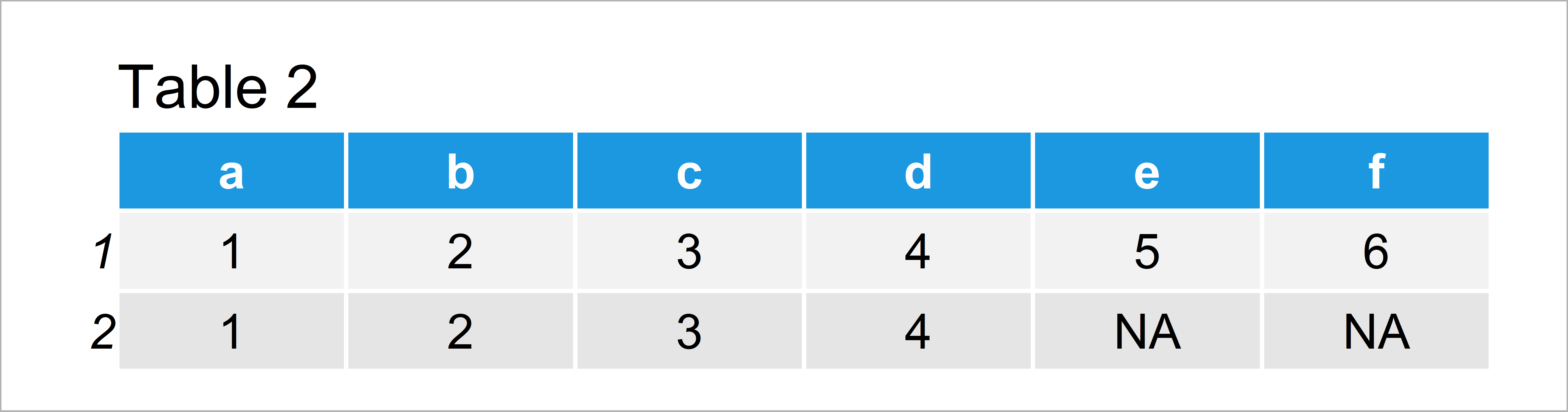# How to cbind & rbind Vectors with Different Length in R (2 Examples)

In this article, I’ll show how to merge multiple vector objects with different length in R programming.

The page will contain two examples for the merging of data. More precisely, the page consists of this information:

Let’s take a look at some R codes in action!

## Creation of Example Data

The data below will be used as basement for this R programming tutorial.

```vec1 <- 1:6                                         # Create first example vector
names(vec1) <- letters[1:6]
vec1                                                # Print first example vector
# a b c d e f
# 1 2 3 4 5 6```

Have a look at the previous output of the RStudio console. It shows that our first example data object is a named vector with a length of six.

Let’s create a second vector object in R:

```vec2 <- 1:4                                         # Create second example vector
names(vec2) <- letters[1:4]
vec2                                                # Print second example vector
# a b c d
# 1 2 3 4```

Our second example vector contains four named vector elements.

## Example 1: Column-Bind Vectors with Different Length Using cbind.na() Function of qpcR Package

In Example 1, I’ll show how to combine two vector objects with different length as columns using the qpcR package.

To be able to use the functions of the qpcR package, we first need to install and load qpcR:

```install.packages("qpcR")                            # Install qpcR package

Next, we can apply the cbind.na function of the qpcR package to combine our two vectors to a matrix:

```data_cbind <- qpcR:::cbind.na(vec1, vec2)           # Bind as columns
data_cbind                                          # Print combined data```Have a look at table 1: It shows that the cbind.na function inserted NA values at the end of the shorter vector.

## Example 2: Row-Bind Vectors with Different Length Using bind_rows() Function of dplyr Package

In this example, I’ll explain how to bind two vectors with different length by rows using the dplyr package.

To be able to use the functions of the dplyr package, we first have to install and load dplyr:

```install.packages("dplyr")                           # Install & load dplyr
library("dplyr")```

Now, we can use the bind_rows function to merge our two vectors by rows:

```data_rbind <- as.data.frame(bind_rows(vec1, vec2))  # Bind as rows
data_rbind                                          # Print combined data```In Table 2 you can see that we have constructed a data frame with two rows using the previously shown R programming code. The shorter vector was set to NA in the later columns.

Note that I have also used the as.data.frame function to create a data frame instead of a tibble. You may remove the as.data.frame function from the previous code in case you prefer to work with tibbles.

## Video, Further Resources & Summary

Do you need more info on the R codes of this tutorial? Then you may watch the following video that I have published on my YouTube channel. In the video, I illustrate the R programming code of this page in RStudio.

Please accept YouTube cookies to play this video. By accepting you will be accessing content from YouTube, a service provided by an external third party.If you accept this notice, your choice will be saved and the page will refresh.

Besides the video, you may want to read the other articles on this homepage. A selection of interesting articles about merging and joining data can be found below:

Subscribe to the Statistics Globe Newsletter

•Gabrielle N.
December 7, 2021 1:45 pm

Content really good and straight forward. Your commands were the only ones that I’ve tried and it worked. Thank you!!

•December 7, 2021 1:59 pm